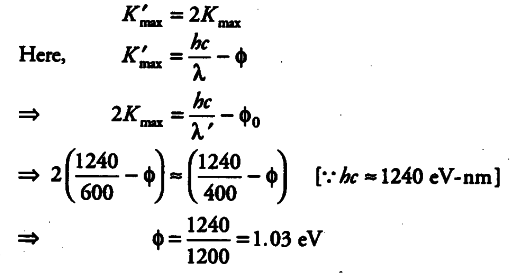# Consider a metal exposed to light of wavelength 600 nm

Consider a metal exposed to light of
wavelength 600 nm. The maximum energy of the electron doubles when light of wavelength 400 nm is used. Find the work function in eV.

Given, for the first condition,
Wavelength of light, λ = 600
Wavelength of light, λ’ = 400 nm
Also, maximum kinetic energy for the second condition is equal to the twice of the kinetic energy in first condition, then,